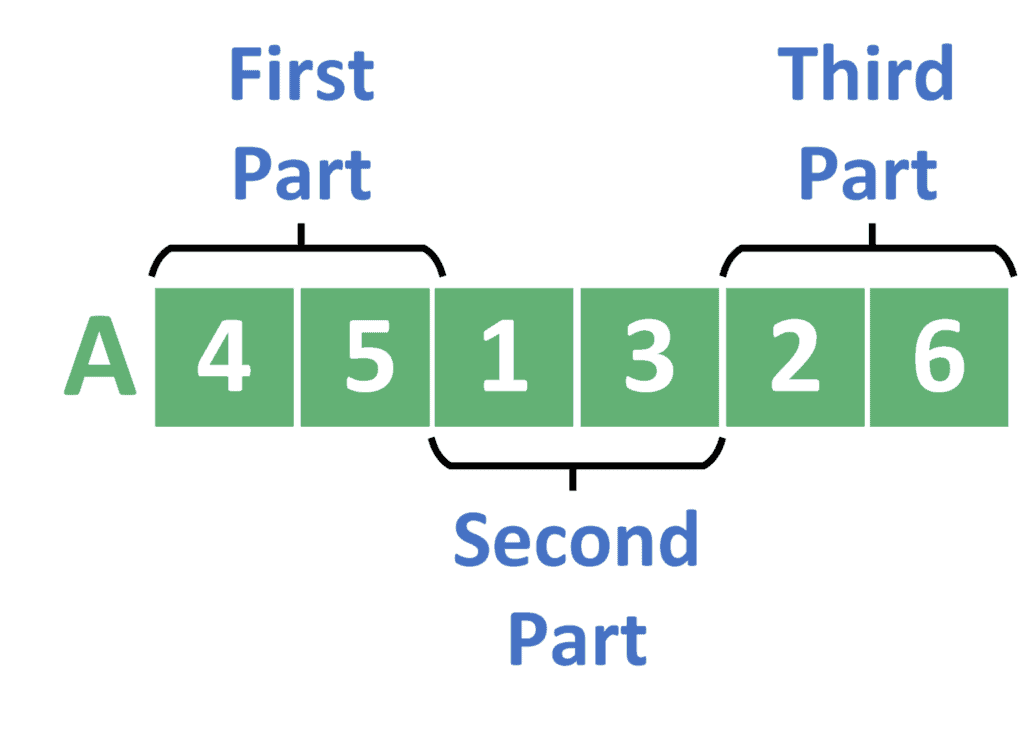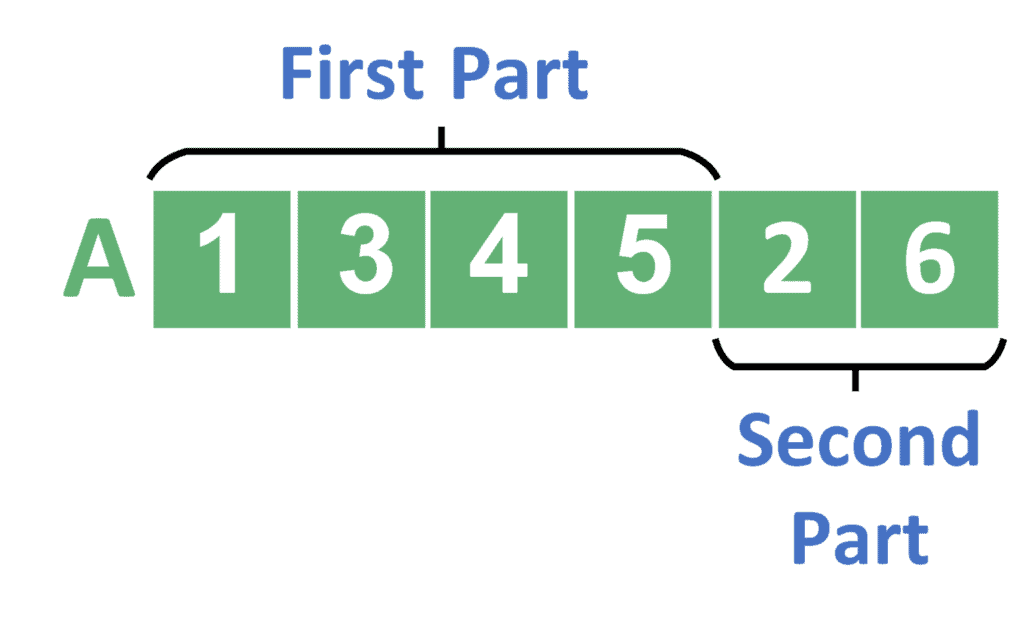## 1. Overview

In this tutorial, we’ll discuss how to implement the merge sort algorithm using an iterative algorithm. First of all, we’ll explain the merge sort algorithm and the recursive version of it.

After that, we’ll discuss the iterative approach of this algorithm. Also, we’ll present a simple example to clarify the idea.

Finally, we’ll present a comparison between the iterative and the recursive algorithms and when to use each one.

## 2. Merge Sort Algorithm

As we know, the merge sort algorithm is an efficient sorting algorithm that enables us to sort an array withintime complexity, whereis the number of values.

Usually, we find that the recursive approach more widespread. Thus, let’s quickly remember the steps of the recursive algorithm so that it’s easier for us to understand the iterative one later. The recursive version is based on the divide and conquers strategy:

• Divide: In this step, we divide the input into two halves, the pivot being the midpoint of the array. This step is carried out recursively for all the half arrays until there are no more halves to divide.
• Conquer: In this step, we sort and merge the divided parts from bottom to top and get the complete sorted result.

## 3. The Iterative Approach

### 3.1. General Idea

As we showed in the recursive version, we divided the input into two halves. This process continued until we got each element of the array alone. Then, we merged the sorted parts from bottom to top until we get the complete result containing all the values sorted.

As usual, when we’re trying to think about moving from a recursive version to an iterative one, we have to think in the opposite way of the recursion. Let’s list a few thoughts that will help us implement the iterative approach:

1. Consider each element of the array as a sorted part. As a start, this part contains a single value.
2. In the second step, merge every two adjacent elements, such that we get sorted parts. In the beginning, each part has two values. However, the last part may contain less than two values if the number of parts was odd.
3. Keep performing steps 1 and 2 until the size of the part reaches the entire array. By then, we can say that the result is sorted.

Now, we can jump into the implementation. However, to simplify the algorithm, we’ll first implement a function responsible for merging two adjacent parts. After that, we’ll see how to implement the complete algorithm based on the merge function.

### 3.2. Merge Function

Let’s implement a simple function that merges two sorted parts and returns the merged sorted array, which contains all elements in the first and second parts. Take a look at the implementation:In the merging function, we use threeloops. The first one is to iterate over the two parts together. In each step, we take the smaller value from both parts and store it inside thearray that will hold the final answer.

Once we add the value to the resulting, we moveone step forward. The variablepoints to the index that should hold the next value to be added to.

In the secondloop, we iterate over the remaining elements from the first part. We store each value inside. In the thirdloop, we perform a similar operation to the secondloop. However, here we iterate over the remaining elements from the second part.

The second and thirdloops are because after the firstloop ends, we might have remaining elements in one of the parts. Since all of these values are larger than the added ones, we should add them to the resulting answer.

The complexity of the merge function is, whereis the length of the first part, andis the length of the second one.

Note that the complexity of this function is linear in terms of the length of the passed parts. However, it’s not linear compared to the full arraybecause we might call the function to handle a small part of it.

### 3.3. Merge Sort

Now let’s use the merge function to implement the merge sort iterative approach. Take a look at the implementation:Firstly, we startfromthat indicates the size of each part the algorithm handles at this step.

In each step, we iterate over all parts of sizeand calculated the beginning and end of each two adjacent parts. Once we determined both parts, we merged them using thefunction defined in algorithm 1.

Note that we handled two special cases. The first one is ifreaches the outside of the array, while the second one is whenreaches the outside. The reason for these cases is that the last part may contain less thanelements. Therefore, we adjust its size so that it doesn’t exceed.

After the merging ends, we copy the elements frominto their respective places in.

Note that in each step, we doubled the length of a single part. The reason is that we merged two parts of length. So, for the next step, we know that all parts of the sizeare now sorted.

Finally, we return the sorted.

The complexity of the iterative approach is, whereis the length of the array. The reason is that, in the firstloop, we double the valuein each step. So, this is. Also, in each step, we iterate over each element inside the array twice and call thefunction for the complete array in total. Thus, this is.

## 4. Example

Let’s take an example to understand the iterative version more clearly. Suppose we have an arrayas follows:Let’s apply the iterative algorithm to.

In the first step, we’ll be merging theelement with theone. As a result, we’ll get a new sorted part which contains the first two values. Since theis smaller than the, we’ll swap both of them.

Similarly, we perform this operation for theandelements. However, in this case, they’re already sorted, and we keep their order. We continue this operation for theandvalues as well.

After these steps,will have its parts of size 2 sorted as follows:In the second step, thebecomes 2. Hence, we have three parts. Each of these parts contains two elements. Similarly to the previous steps, we have to merge the first and the second parts. Thus, we get a new part that contains the first four values. For the third part, we just skip it because it was sorted in the previous step, and we don’t have a fourth part of merging it with.

Take a look at the result after these steps:In the last step, thebecomes 4, and we have two parts. The first one contains four elements, whereas the second one contains two. We call the merging function for these parts.

After the merging ends, we get the final sorted answer:## 5. Comparison

As usual, recursion reduces the size of the code, and it’s easier to think about and implement. On the other hand, it takes more memory because it uses the stack memory which is slow in execution.

For that, we prefer to use the iterative algorithm because of its speed and memory saving. However, if we don’t care about execution time and memory as if we have a small array, for example, we can use a recursive version.

## 6. Conclusion

In this tutorial, we explained how to implement the merge sort algorithm using an iterative approach. Firstly, we discussed the merge sort algorithm using the recursive version of the algorithm.

Then, we discussed the iterative version of this algorithm. Also, we explained the reason behind its complexity.

After that, we provided a simple example to clarify the idea well.

Finally, we presented a comparison between the iterative and the recursive algorithms and showed the best practice of using each of them.